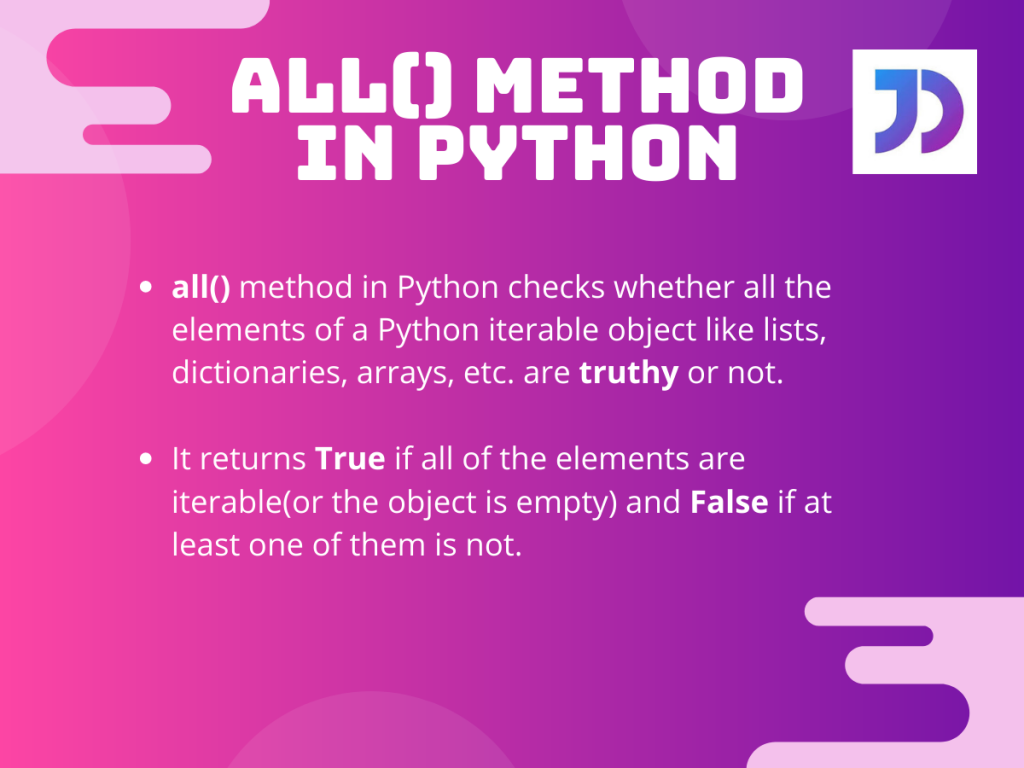# all() Method In Python ProgrammingPython comes with many interesting pre-defined methods. One of them is the `all()` method in Python. This method is widely used to check whether all the elements of an iterable Python object are truthy. So let us learn more about the `all()` method and also take a look at how we can incorporate in our code.

## The Working of all() Method in Python

Theoretically, the all() method in Python checks whether all the elements of a Python iterable object like lists, dictionaries, arrays, etc. are truthy or not. It returns `True` if all of the elements are iterable( or the object is empty) and `False` if at least one of them is not.

Did you notice that we use the term “Truthy” and not “True”? This is because both the terms have different meanings.

In Python, `all()` checks for all the elements if `bool(element)` is true or not. In that way, we can infer that truthy is actually different than true here.

## all() Function Usage And Examples

Now let’s take a look at an example that can illustrate the working of the `all()` method in Python.

```#python all() example
print("all() in Python:")

#Defining different type of variables
list1=['J','o','u','r','n','a','l','D','e','v']
list2=[0,1,1,1,0]
dictionary1={1:"True",2:"False"}
dictionary2={0:"False",1:"True"}
tpl1=(0,1,2,3)

#Evaluating the variables with the all() method.
print("list1=['J','o','u','r','n','a','l','D','e','v']:",all(list1))
print("list2=[0,1,1,1,0]:",all(list2))
print("tpl1=(0,1,2,3):",all(tpl1))
print("dictionary1:",all(dictionary1))
print("dictionary2:",all(dictionary2))

# Testing all() method's evaluation of empty objects
print("Now for empty objects:")
dict_empt={}
list_empt=[]
print("dict_empt:",all(list_empt))
print("list_empt:",all(dict_empt))
```

Output:

• For list1, `all()` returns `True` as all of its elements are non-falsy,
• Whereas, for list2 we got `False` because it contains the number 0 which evaluates to false.
• For tuple tpl1, also the method returns `False` since the first element is 0 which evaluates to false.
• For dictionary1, we get `True` as the output as none of the keys are 0 or false. For demonstration purposes, we added the “False” string which evaluates to TRUE since the false string is not a boolean FALSE.
• We get a `False` for dictionary2 as one of its keys is 0.
• For any kind of empty iterable object, be it list or dictionary, the `all()` method returns `True`.